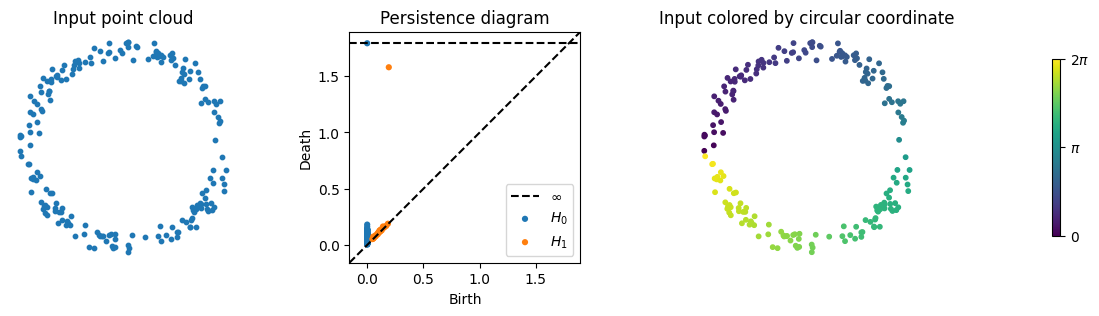DREiMac: Dimensionality reduction with Eilenberg-MacLane coordinates

# DREiMac: Dimensionality Reduction with Eilenberg-MacLane Coordinates

DREiMac is a library for topological data coordinatization, visualization, and dimensionality reduction. Currently, DREiMac is able to find topology-preserving representations of point clouds taking values in the circle, in higher dimensional tori, in the real and complex projective space, and in lens spaces.

In a few words, DREiMac takes as input a point cloud together with a topological feature of the point cloud (in the form of a persistent cohomology class), and returns a map from the point cloud to a well-understood topological space (a circle, a product of circles, a projective space, or a lens space), which preserves the given topological feature in a precise sense. For more information, please check the theory and examples in the documentation.

## Basic usage

Here is a simple example; please check the Jupyter notebooks in the notebooks directory for further examples.

# basic imports
from dreimac import CircularCoords
from persim import plot_diagrams
import matplotlib.pyplot as plt
import numpy as np

# prepare plot with 4 subplots
f, (a0, a1, a2, a3) = plt.subplots(1, 4, width_ratios=[1, 1, 1, 0.2], figsize=(14,3))

# 200 samples from a noisy circle
n_samples = 200
np.random.seed(0)
Z = np.random.random((n_samples, 2)) - 0.5
X = Z / np.linalg.norm(Z, axis=1).reshape((n_samples, 1)) + (np.random.random((n_samples, 2)) - 0.5) * 0.2

# plot point cloud
a0.scatter(X[:,0], X[:,1], s=10)
a0.set_title("Input point cloud") ; a0.axis("off") ; a0.set_aspect("equal")

# plot the persistence diagram, showing a single prominent class
cc = CircularCoords(X, n_landmarks=200)
plot_diagrams(cc._dgms, title="Persistence diagram", ax=a1)

# plot the data colored by the circle-valued map constructed by DREiMac
circular_coordinates = cc.get_coordinates()
a2.scatter(X[:,0], X[:,1], c=circular_coordinates, s=10, cmap="viridis")
a2.set_title("Input colored by circular coordinate") ; a2.axis("off") ; a2.set_aspect("equal")

# plot colorbar
img = a3.imshow([[0,1]], cmap="viridis"); a3.set_visible(False)
cb = plt.colorbar(mappable=img,ticks=[0,0.5,1]) ; _ = cb.ax.set_yticklabels(["0","$\pi$","2$\pi$"])## More examples

For Jupyter notebooks with more examples, please check the documentation or this repository's docs/notebooks directory.

## Installing

Make sure your Python version is >=3.8 and <3.12. DREiMac depends on the following python packages, which will be installed automatically when you install with pip: matplotlib, numba, numpy, persim, ripser, and scipy.

To install the latest release:

pip install dreimac


To install directly from GitHub:

pip install git+https://github.com/scikit-tda/DREiMac.git


## Documentation and support

You can find the documentation here, including the API reference. If you have further questions, please open an issue and we will do our best to help you. Please include as much information as possible, including your system's information, warnings, logs, screenshots, and anything else you think may be of use.

## Running the tests

If you want to check that your machine is running DREiMac properly, you may run the tests by running the following commands from the root directory of a clone of this repository.

pip install pytest
pip install -r requirements.txt
pytest .


## Contributing

To contribute, you can fork the project, make your changes, and submit a pull request. If you're looking for a way to contribute, you could consider:

• adding documentation to existing functionality;
• adding missing tests to improve coverage;
• adding a Jupyter notebook with a tutorial or demo;
• adding functionality and the corresponding documentation and tests;
• responding to a bug or feature request in the Github issues.

## Authors

Jose A. Perea, Luis Scoccola, Chris Tralie

## Acknowledgements

We thank Tom Mease for contributions and discussions.

This software is published under Apache License Version 2.0.

## Project details

This version0.3.10.3.00.2.20.2.00.1

Uploaded source
Uploaded py3